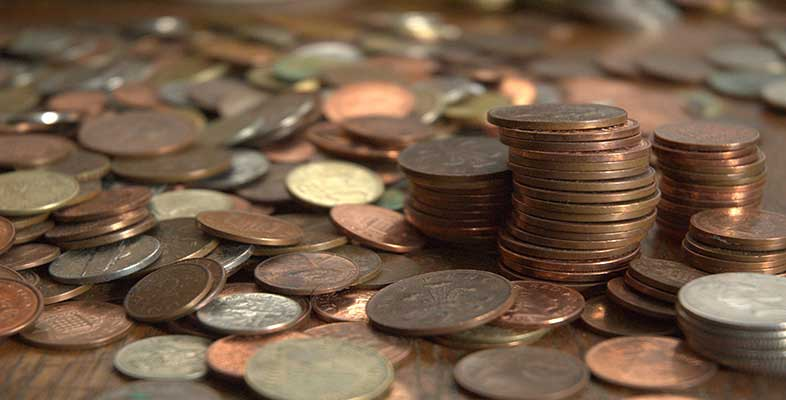### Become an OU studentIntroduction to bookkeeping and accounting

Start this free course now. Just create an account and sign in. Enrol and complete the course for a free statement of participation or digital badge if available.

# 1.6 Percentages

Percentages also indicate proportions. They can be expressed either as fractions or as decimals:

45% = 45/100 = 0.45

7% = 7/100 = 0.07

Their unique feature is that they always relate to a denominator of 100. Percentage means simply ‘out of 100’ so 45% is ‘45 out of 100’, 7% is ‘7 out of 100’, etc.

A company is offered a loan to a maximum of 80% of the value of its premises. If the premises are valued at £120,000 then the company can borrow the following:

£120,000 x 80% = £120,000 x 0.80 = £96,000

Fractions and decimals can also be converted to percentages. To change a decimal to a percentage you need to multiply by 100:

0.8 = 80%

0.75 = 75%

To change a percentage to a decimal you need to divide by 100:

60% = 0.6

3% = 0.03

To convert a fraction to a percentage it is necessary to first change the fraction to a decimal:

4/5 = 0.8 = 80%

3/4 = 0.75 = 75%

If a machine is sold for £120 plus VAT (Value Added Tax – a sales tax in the UK) at 17.5% then the actual cost to the customer is:

£120 + (17.5% of 120) = £120 + (0.175 x 120) = £141

Alternatively, the amount can be calculated as:

£120 x (100% + 17.5%) = £120 x (1.00 + 0.175) = £120 x 1.175 = £141

If the machine were quoted at the price that included VAT (the gross price), and we wanted to calculate the price before VAT, then we would need to divide the amount by (100% + 17.5%) = 117.5% or 1.175. The gross price of £141 divided by 1.175 would thus give the net price of £120. This principle can be applied to any amount which has a percentage added to it.

For example, a restaurant bill is a total of £50.40 including a 12% service charge. The bill before the service charge was added would be:

£50.40 / 1.12 = £45.00

## Activity 8

### Part (a - i)

(a) Convert the following to percentages

(i) 0.9

(i) 90%

(ii) 1.2

(ii) 120%

(iii) 1/3

(iii) 33.33%

(iv) 0.03

(iv) 3%

(v) 1/10

(v) 10%

### Part (a - vi)

(vi) 1 1/4

(vi) 125%

(b) A company sells its product for £65 per unit. How much will it sell for if the customer negotiates a 20% discount?